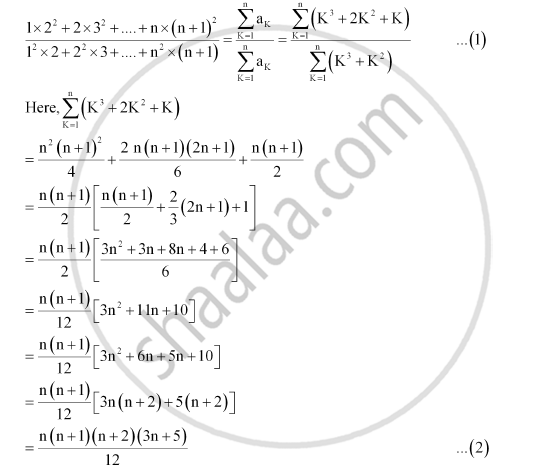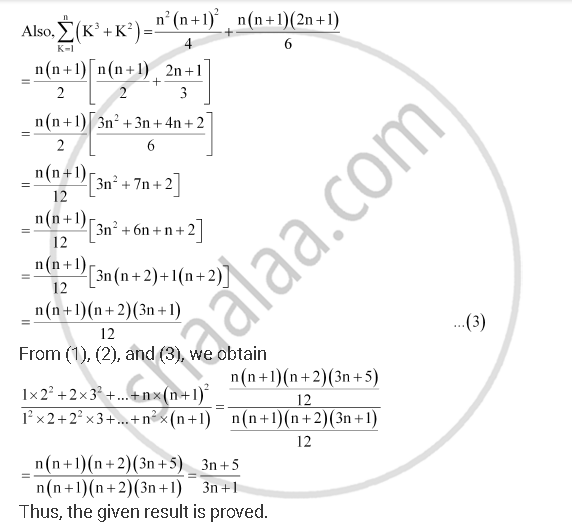CBSE (Arts) Class 11CBSE
Share
Notifications

View all notifications
Books Shortlist
Your shortlist is empty

# Show that (1xx2^2 + 2xx3^2 + ...+Nxx(N+1)^2)/(1^2 Xx 2 + 2^2 Xx3 + ... + N^2xx (N+1)) = (3n + 5)/(3n + 1) - CBSE (Arts) Class 11 - Mathematics

Login
Create free account

Forgot password?
ConceptSum to N Terms of Special Series

#### Question

Show that  (1xx2^2 + 2xx3^2 + ...+nxx(n+1)^2)/(1^2 xx 2 + 2^2 xx3 + ... + n^2xx (n+1)) = (3n + 5)/(3n + 1)

#### Solution

nth term of the numerator = n(n + 1)2 = n3 + 2n2 + n

nth term of the denominator = n2(n + 1) = n3 + n2Is there an error in this question or solution?

#### APPEARS IN

NCERT Solution for Mathematics Textbook for Class 11 (2018 to Current)
Chapter 9: Sequences and Series
Q: 26 | Page no. 200
Solution Show that (1xx2^2 + 2xx3^2 + ...+Nxx(N+1)^2)/(1^2 Xx 2 + 2^2 Xx3 + ... + N^2xx (N+1)) = (3n + 5)/(3n + 1) Concept: Sum to N Terms of Special Series.
S